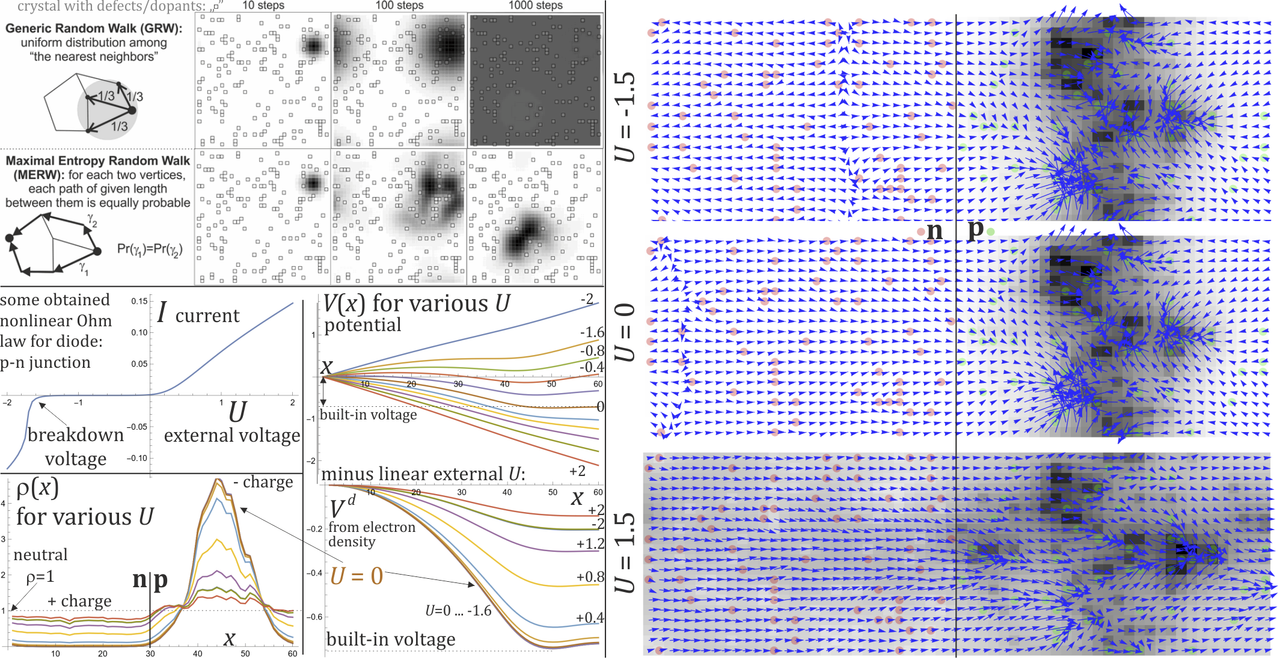# Diffusion model of p-n junction (diode) - conductance prevented by Anderson localization

## Recommended Posts

Just prepared: https://arxiv.org/pdf/2112.12557

While textbook explanation of p-n junction ( https://en.wikipedia.org/wiki/P–n_junction ) looks quite heuristic, this is just using statistical mechanics - no "holes", only electron dynamics.

Lattice 60x30 atoms below, dopants of different potentials are presented as red/green dots, grayness shows calculated electron densities, arrows show local currents.
The model is:
- use 3 types of potentials: of individual atoms + from external voltage + mean-field self interaction (from found charge density),
- apply entropy maximizing diffusion ( https://en.wikipedia.org/wiki/Maximal_entropy_random_walk ),
getting e.g. below diagrams.

Are there there others atomic-scale conductance models?
What applications could they have, e.g. some technology optimization?Edited by Duda Jarek

## Create an account

Register a new account